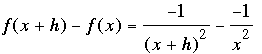SEARCH HOMEMath Central Quandaries & QueriesQuestion from Marie, a student: For each function defined as follows, find a.) f (x+h) b. f(x+h)-f(x) c. [f(x+h)- f(x)]/h. Problem: f(x)= -1/ x^2. Help me solve part b and cMarie,

Try some numbers first.

• If x = 3 then f(x) = f(3) = -1/32 = -1/9.

• If x = 3 + 5 then f(x) = f(3 + 5) = -1/(3 + 5)2 = -1/82 = -1/64.

• If x = 3 + h then f(x) = f(3 + h) = -1/(3 + h)2.

I hope now you see that f(x + h) = -1/(x + h)2.

Thus for b,. Write the two fractions with a common denominator and then simplify the numerator.

Write back and tell me what you got,
HarleyMath Central is supported by the University of Regina and The Pacific Institute for the Mathematical Sciences.